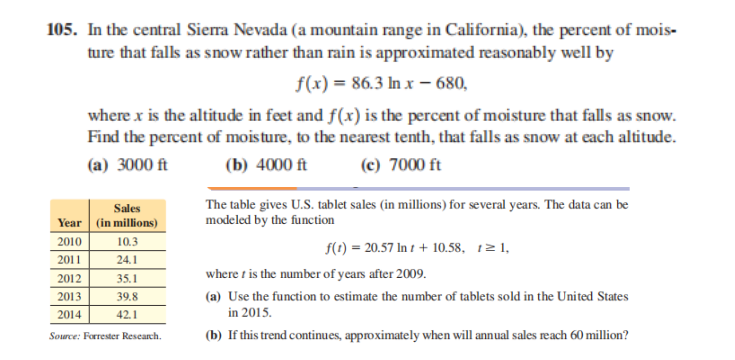### Still have math questions?

Algebra
QuestionIn the central Sierra Nevada (a mountain range in California), the percent of mois- ture that falls as snow rather than rain is approximated reasonably well by

$$f ( x ) = 86.3 \ln x - 680$$ , where $$x$$ is the altitude in feet and $$f ( x )$$ is the percent of moisture that falls as snow. Find the percent of moisture, to the nearest tenth, that falls as snow at each altitude. (a) $$3000 ft$$ (b) $$4000 ft$$ (c) $$7000 ft$$

The table gives U.S. tablet sales (in millions) for several years. The data can be modeled by the function $$f ( t ) = 20.57 \ln t + 10.58 , t \geq 1$$ ,